本项目拟通过该数据集的探索，结合自己的理解进行分析，最终目的的是初步预测哪些人贷款后会还款、哪些人会赖账。

1.探索数据集

``` loandata = read.csv("prosperLoanData.csv")
str(loandata)```

结论:一共有81个变量,113937个对象

2.选择分析的变量

```由于有81个变量，为简化分析，仅选取其中有代表性的13个变量。变量选取如下：

1. **ListingCreation**: 列表创建时间。

2. **Term**: 贷款期限，期限越长的，利率应当越高

3. **LoanStatus**: 贷款状态（Completed、Current、Defaulted、Chargedoff等）

4. **BorrowerBorrowerRate**: 借款利率。

5. **DelinquenciesLast7Years**: 信用资料提交时借款人过去7年违约次数。

6. **StatedMonthlyIncome**: 客户自己声称的月收入。

7. **IsBorrowerHomeowner**: 借款人是否拥有住房。

8. **InquiriesLast6Months**: 最近6个月的征信记录查询次数。一般来说，征信查询次数越多，该主体的贷款申请就越多。

9. **DebtToIncomeRatio**: 借款人的债务收入比，债务收入比越高说明筹资者财务状况越差，还款能力越低。

10. **Occupation**: 贷款人职业

12. **CreditScore**: 由消费信用公司提供的消费信用评分，同信用评级作用。

13. **BankCardUse**: 信用资料提交时借款人信用卡使用额度和信用卡总透支额度的百分比，本文将这个数据分成四组（mild use; medium use; heavy use; super use）```

3.数据预处理

3.1贷款状态

`# 查看贷款状态1 table(loandata\$LoanStatus)`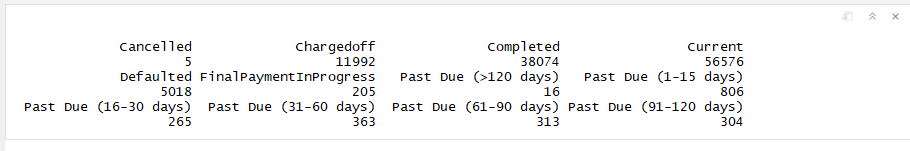``` # 2.整合数据 # 针对LoanStatus进行重构 # 1.将所有含有"Past Due"字段的值统一为"PastDue" # 2.将"Cancelled"归类到"Current"中 # 3.将"defaulted"归类到"Chargedoff"中 # 4.将"FinalPaymentInProgress"归类到"Completed"中 1 PastDue <- c("Past Due (>120 days)",
"Past Due (1-15 days)",
"Past Due (16-30 days)",
"Past Due (31-60 days)",
"Past Due (61-90 days)",
"Past Due (91-120 days)")
loandata\$LoanStatus <- as.character(loandata\$LoanStatus)
loandata\$LoanStatus[loandata\$LoanStatus %in% PastDue] <- "PastDue"
loandata\$LoanStatus[loandata\$LoanStatus == "Cancelled"] <- "Current"
loandata\$LoanStatus[loandata\$LoanStatus == "Defaulted"] <- "Chargedoff"
loandata\$LoanStatus[loandata\$LoanStatus == "FinalPaymentInProgress"] <- "Completed"`````` # 3.再次整合数据
# 1.然后将"PastDue"与"Chargedoff"合并，表示未按时还款
# 2.将"Current"和"Completed"合并，表示按时还款未出现不良记录的
loandata\$LoanStatus[loandata\$LoanStatus == "PastDue"] <- "Chargedoff"
loandata\$LoanStatus[loandata\$LoanStatus == "Current"] <- "Completed"
table(loandata\$LoanStatus)```3.2消费信用评分

``` # 对借款人的消费信用评级，数据中有高低范围，将这两个数值取平均值做计算
loandata\$CreditScore <- (loandata\$CreditScoreRangeLower + loandata\$CreditScoreRangeUpper) / 2```

3.3信用评级

``` # 因2009年7月1日，Prosper的信用评级模型发生了改变，所以需要进行分段处理

# 先处理2009年7月1日前的数据
levels = c("NC", "HR", "E", "D", "C", "B", "A", "AA"))
# 再处理2009年7月1日后的数据
loandata\$ProsperRating..Alpha. <- ordered(loandata\$ProsperRating..Alpha.,
levels = c("HR", "E", "D", "C", "B", "A", "AA"))
# 因为2009年7月1日是一个数据节点，因此将数据分成两段处理
loandata\$LoanOriginationDate <- as.Date(loandata\$LoanOriginationDate)
loandata\$Phase[loandata\$LoanOriginationDate > "2009-07-01"] <- "After 2009"
loandata\$Phase[loandata\$LoanOriginationDate < "2009-07-01"] <- "Before 2009"
table(loandata\$Phase)```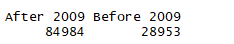3.4信用卡使用程度

``` #定义"Mild Use"
loandata\$BankCardUse[
loandata\$BankcardUtilization < quantile(loandata\$BankcardUtilization,
probs = 0.25, "na.rm" = TRUE)
] <- "Mild Use"

#定义“Medium Use”
loandata\$BankCardUse[
loandata\$BankcardUtilization >= quantile(loandata\$BankcardUtilization,
probs = 0.25, "na.rm" = TRUE)
&
loandata\$BankcardUtilization < quantile(loandata\$BankcardUtilization,
probs = 0.5, "na.rm"= TRUE)
] <- "Medium Use"

#定义“Heavy Use”
loandata\$BankCardUse[
loandata\$BankcardUtilization >= quantile(loandata\$BankcardUtilization,
probs = 0.5, "na.rm" = TRUE)
&
loandata\$BankcardUtilization < quantile(loandata\$BankcardUtilization,
probs = 0.75, "na.rm" = TRUE)
] <- "Heavy Use"

#定义“Super Use”
loandata\$BankCardUse[loandata\$BankcardUtilization >= 0.75] <- "Super Use"

loandata\$BankCardUse <- factor(loandata\$BankCardUse,
levels = c("Mild Use",
"Medium Use",
"Heavy Use",
"Super Use"))

table(loandata\$BankCardUse)```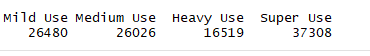4.探索数据集

4.1单变量探索

4.1.1贷款期限

``` ggplot(aes(x = as.factor(Term)), data = loandata) +
geom_histogram(stat = "count") +
xlab("Term")
table(loandata\$Term, dnn = ("Term"))```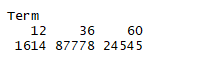结论:一年期（12个月）的有1614笔，三年期（36个月）的有87778笔，五年期（60个月）的有24545笔。由此，平台上的贷款以中长期为主，且三年期占绝大多数。经查阅资料发现，Prosper平台是　　　　　　　　　　2009年以后才开始提供一年期和五年期贷款品种。

4.1.2贷款利率

```ggplot(aes(x = BorrowerRate), data = loandata) +
geom_histogram(binwidth = 0.01) +
scale_x_continuous(breaks = seq(0, 0.5, 0.05)) +
facet_wrap(~ Term, ncol = 3)```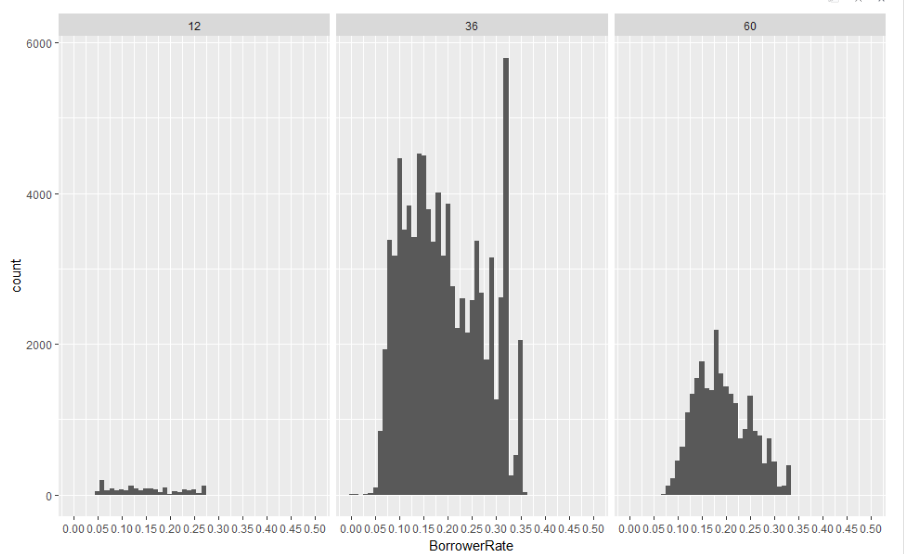结论:平台上的借款利率多集中在0.08至0.2这个区间，另外，利率0.32附近也拥有大量的观测值。并且贷款利率似乎与贷款期限关系并不明显，未如经验判断的“期限越长、利率越高”。

4.1.3过去7年的违约次数

``` ggplot(aes(x = DelinquenciesLast7Years), data = loandata) +
geom_density(aes(fill = I("grey"))) +
coord_cartesian(xlim = c(0, quantile(loandata\$DelinquenciesLast7Years,
probs = 0.95, "na.rm" = TRUE)))```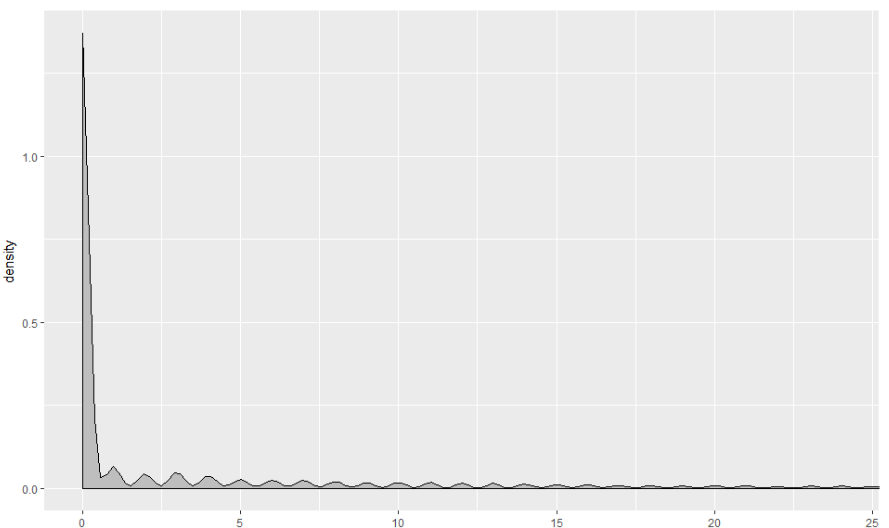结论:大部分借款人在过去7年内的违约次数均为0，这说明整个平台借款人的信用状况较好

4.1.4月收入,月收入很大情况下能够反映借贷人的还款能力

``` ggplot(aes(x = StatedMonthlyIncome),
data = subset(loandata, loandata\$StatedMonthlyIncome <
quantile(loandata\$StatedMonthlyIncome,
probs = 0.95, "na.rm" = TRUE))) +
geom_histogram(binwidth = 500) +
scale_x_continuous(breaks = seq(0, 21000, 500)) +
geom_vline(xintercept = 2500, color = "red") +
geom_vline(xintercept = 6000, color = "red")```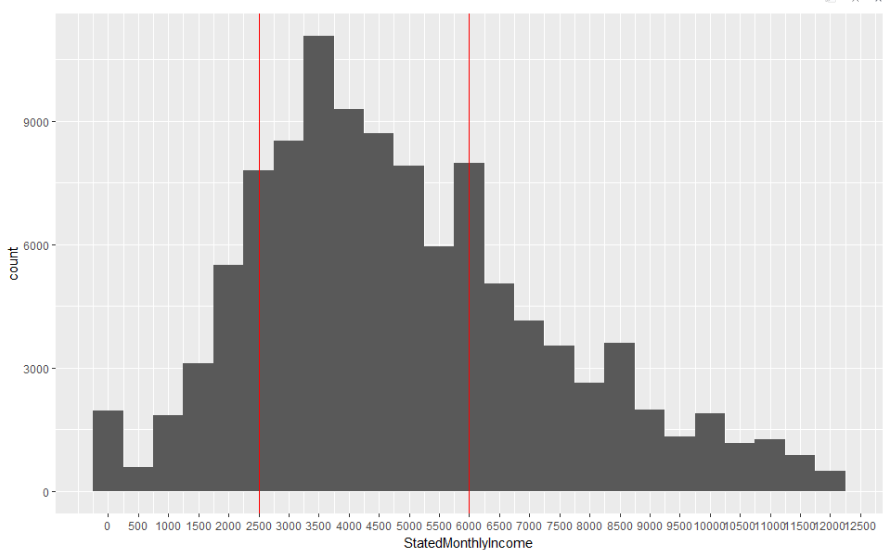结论:大部分借贷人的月薪在2500~6000美金之间

4.1.5是否拥有房屋(有房子的要比没有房屋的人偿还能力强)

``` ggplot(aes(x = IsBorrowerHomeowner), data = loandata) +
geom_bar()```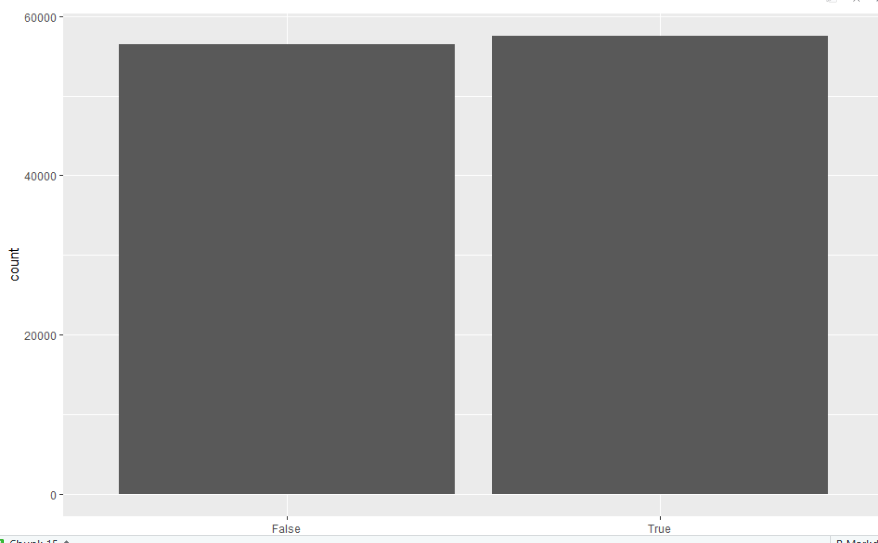结论:有房屋的借贷人略微大于没房屋的借贷人

4.1.6借款人征信查询的次数,征信查询的次数越多表示借款人近期的申请的贷款越多,资金越紧张

```ggplot(aes(x = InquiriesLast6Months, fill = I("grey")), data = loandata) +
geom_density(binwidth = 1) +
geom_vline(xintercept = quantile(loandata\$InquiriesLast6Months,
probs = 0.95, "na.rm" = TRUE),
linetype = "dashed", color = "red") +
coord_cartesian(xlim = c(0, quantile(loandata\$InquiriesLast6Months,
probs = 0.95, "na.rm" = TRUE)))```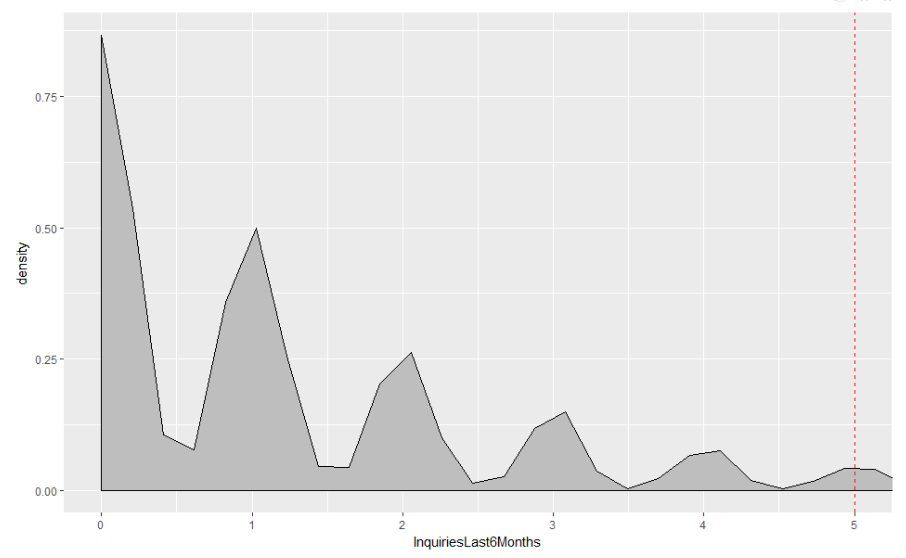结论:95%的借款人征信查询次数在5次及以下

4.1.7负债收益比(反映了借款人的杠杆率,如果大于1表示很难偿还贷款,极易引发坏账)

``` ggplot(aes(x = DebtToIncomeRatio), data = loandata) +
geom_histogram(binwidth = 0.05) +
scale_x_continuous(breaks = seq(0, 10, 0.5)) +
geom_vline(xintercept = quantile(loandata\$DebtToIncomeRatio,
probs = 0.95, "na.rm" = TRUE),
linetype = "dashed", color = "red")```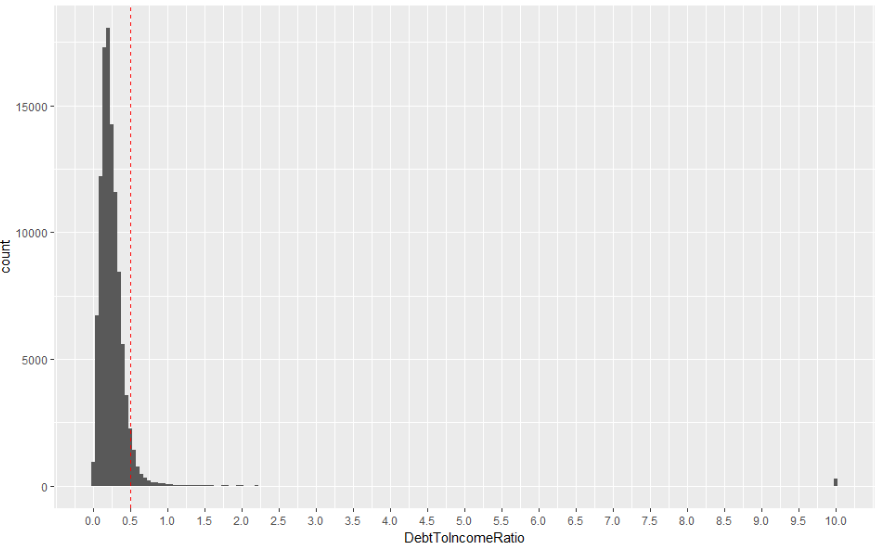结论:95%的借款人负债收入比小于0.5，另外注意到，极少数人的负债收入比达到甚至超过10,总体来说平台的负债收益比较低,资质较好

4.1.8借款人职业

```data_count <- loandata %>%
group_by(Occupation) %>%
summarise(n = n())

ggplot(aes(x = reorder(Occupation, -n), y = n),
data = data_count) +
geom_bar(stat = "identity") +
theme(axis.text.x = element_text(angle = 90,
vjust = 0.5,
hjust = 1))```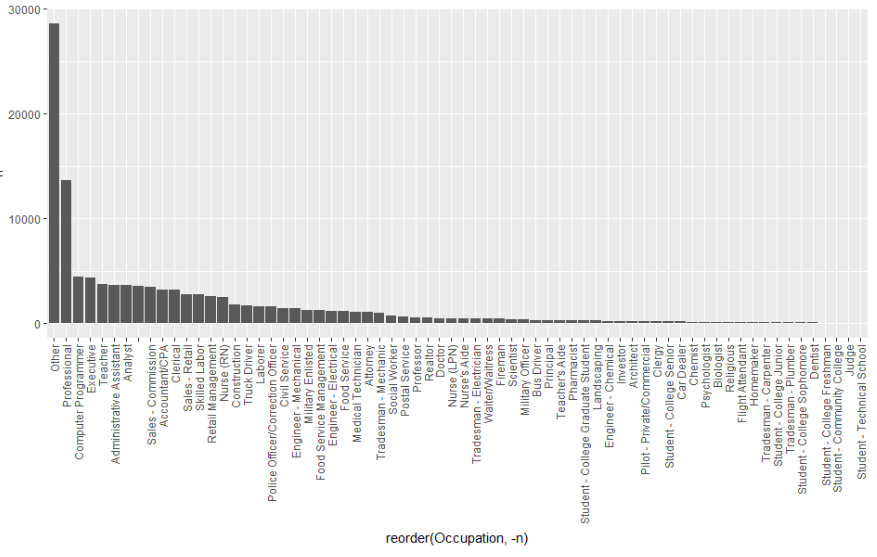结论:职业中，选择“other”的人数最多，说明很多人在申请贷款时出于隐私或者其他原因不选择职业，该数据的真实性存疑

4.1.9贷款状态

```ggplot(aes(x = LoanStatus), data = loandata) +
geom_bar()
table(loandata\$LoanStatus)```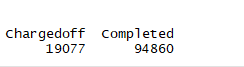结论:Prosper平台整体贷款的违约率约为16.74%

4.1.10贷款日期

``` loandata\$ListingCreationDate <- as.Date(loandata\$ListingCreationDate)

ggplot(aes(x = ListingCreationDate), data = loandata) +
geom_histogram()```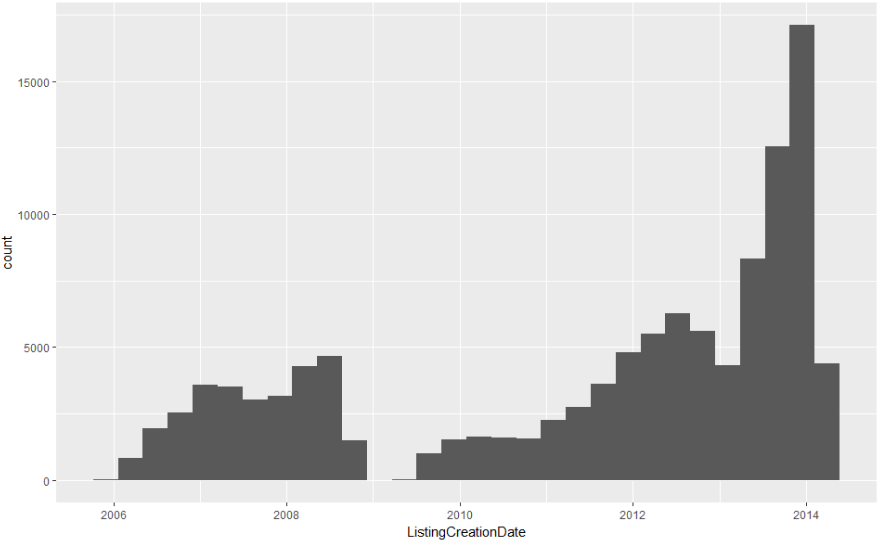结论:09年前后，平台贷款成交量几乎为0，通过查阅相关资料发现，Prosper平台因涉诉被迫于2008年11月24日暂停运营，并于2009年7月1日重新开始营运。

4.1.11客户信用评价

``` p1 <- ggplot(aes(x = CreditScore), data = loandata) +
geom_histogram()+
coord_cartesian(xlim = c(400, 900)) +
scale_x_continuous(breaks = seq(400, 900, 50)) +
ggtitle("The Histogram of Borrowers' CreditScore")

data = subset(loandata, LoanOriginationDate < "2009-07-01")) +
geom_bar() +
scale_x_discrete(limits = c("HR","E","D","C","B","A","AA")) +

p3 <- ggplot(aes(x = ProsperRating..Alpha.),
data = subset(loandata, LoanOriginationDate > "2009-07-01")) +
geom_bar() +
scale_x_discrete(limits = c("HR","E","D","C","B","A","AA")) +

grid.arrange(p1, p2, p3, ncol = 1)```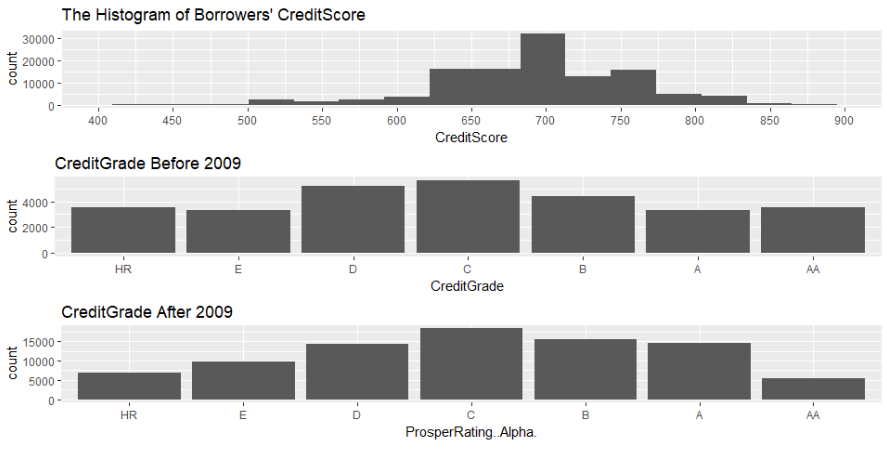结论:大部分借款人的消费信用评分都高于600分，集中于700分左右，而2009年前后的信用评级也显示出较为优秀的信用资质B级。
4.1.12信用卡使用情况

``` ggplot(aes(BankCardUse),
data = subset(loandata, !is.na(BankcardUtilization))) +
geom_bar()```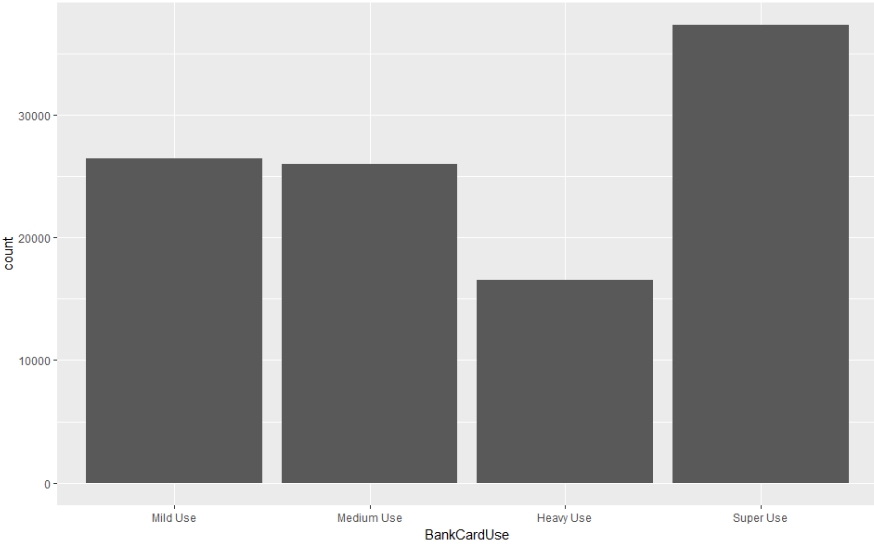结论:大部分人都处于Super Use状态，借款人的还款能力会因此而打折扣

单变量分析总结论:

```数据集中共含有113937笔贷款记录，每笔记录均有12个特征描述，分别是：贷款日期（ListingCreationDate）、贷款期限（Term）、贷款状态（LoanStatus）、贷款利率（BorrowerRate）、借款人过去7年违约次数（DelinquenciesLast7Years）、借款人月收入（StatedMonthlyIncome）、借款人是否拥有房屋（IsBorrowerHomeowner）、借款人最近6个月的征信查询次数（InquiriesLast6Months）、借款人负债收入比（DebtToIncomeRatio）、借款人职业（Occupation）、借款人信用评价（CreditScore / CreditGrade / ProsperRating(Alpha)）及借款人的信用卡使用情况（BankCardUse）。

1. 大部分贷款的期限为三年期。

2. 整个平台贷款的违约率为16.74%。

3. 大部分贷款利率集中在0.08至0.2这个区间，0.32附近也有较大的成交量。

4. 平台上的借款人整体信用资质较好。

5. 从信用卡使用角度来看，借款人的还款压力较大。

6. 大部分借款人的月收入集中在2500至6000美元之间。

7. 拥有房屋的借款人与未拥有房屋的借款人数量大致相当。

8. 出于隐私或者其他原因，借款人申请贷款时职业一项选择“other”的极多。```

4.2双变量探索

4.2.1贷款利率随着时间变化的趋势

```ggplot(aes(x = ListingCreationDate, y = BorrowerRate), data = loandata) +
geom_point(alpha = 0.05) +
facet_wrap(~Term)```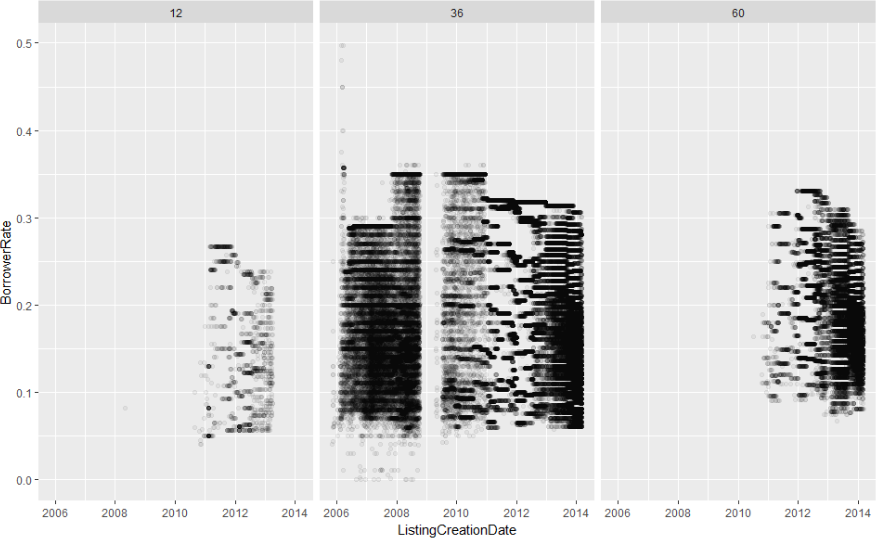结论:一年期与五年期贷款是在2011年前后才上线的产品。三年期是整个平台最主要的贷款,

2008年以前，贷款利率主要分布在0.08至0.2这个区间，随着美国次贷危机的持续发酵2010年利率逐渐分化，优质借款人依旧可以拿到0.1甚至更低的利率，而更多的借款人则需要以0.2以上甚至　　　　　　　　　　   0.35的利率方可筹到资金。为了救市美联储实行了量化宽松政策，持续向市场中注入大量流动性，利率承压下行。至2014年，可以看到各个利率层面均有大量的成交记录信贷市场逐渐恢复元气。

4.2.2贷款利率与贷款状态

``` ggplot(aes(x = LoanStatus, y = BorrowerRate), data = loandata) +
geom_boxplot()```结论:违约贷款组的利率明显高于正常还款组。一方面，贷款定价高是由于借款人自身资质差；另一方面，高利率又使得借款人更加难以承受进而违约

4.2.3贷款利率与过去7年违约次数

``` ggplot(aes(x = DelinquenciesLast7Years, y = BorrowerRate),
data = subset(loandata,
loandata\$DelinquenciesLast7Years <
quantile(loandata\$DelinquenciesLast7Years,
probs = 0.95, "na.rm" = TRUE))) +
geom_point(alpha = 0.05, position = "jitter") +
geom_smooth()```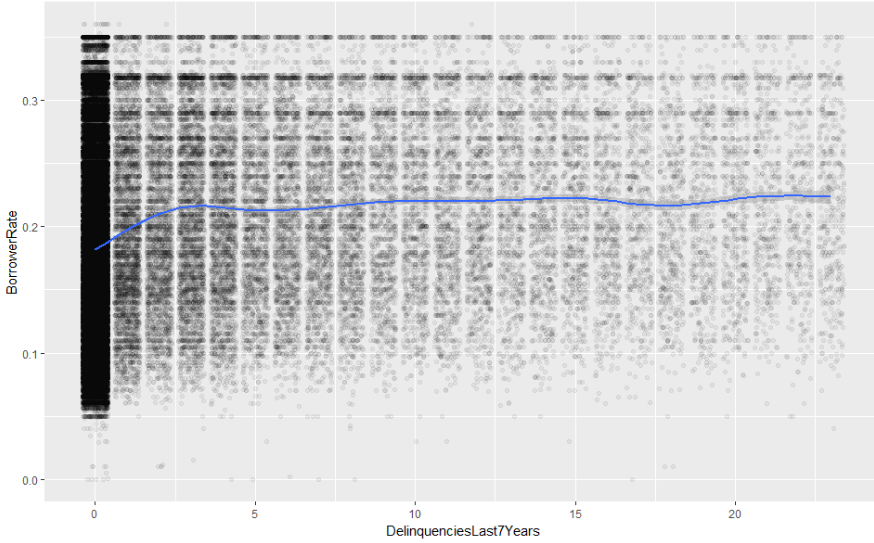结论:95%的借款人过去7年违约次数低于23次，违约次数低于3次时，违约次数与贷款利率呈现出弱正相关性；而3次以后两者关系就趋于平稳。

4.2.4贷款利率和借款人月收入的关系

``` ggplot(aes(x = StatedMonthlyIncome, y = BorrowerRate),
data = subset(loandata, !is.na(loandata\$StatedMonthlyIncome))) +
stat_density2d(aes(alpha = ..density..), geom = "tile", contour = FALSE) +
scale_x_continuous(limits = c(0, 30000))```结论:平台的借贷人月收入在10000美金以下,未发现贷款利率和月收入存在关系

4.2.5贷款利率与借款人是否拥有房屋之间的关系

``` ggplot(aes(x = BorrowerRate), data = loandata) +
geom_histogram() +
geom_hline(yintercept = 3000, linetype = "dashed", color = "red") +
facet_wrap(~IsBorrowerHomeowner, ncol = 1)```结论:拥有房屋的人比没有房屋的人贷款的利息更低

4.2.6贷款利率与过去6个月征信查询次数的关系

``` ggplot(aes(x = as.factor(InquiriesLast6Months), y = BorrowerRate),
data = subset(loandata, loandata\$InquiriesLast6Months <
quantile(loandata\$InquiriesLast6Months,
probs = 0.95, "na.rm" = TRUE))) +
geom_boxplot() +
xlab("InquiriesLast6Months")```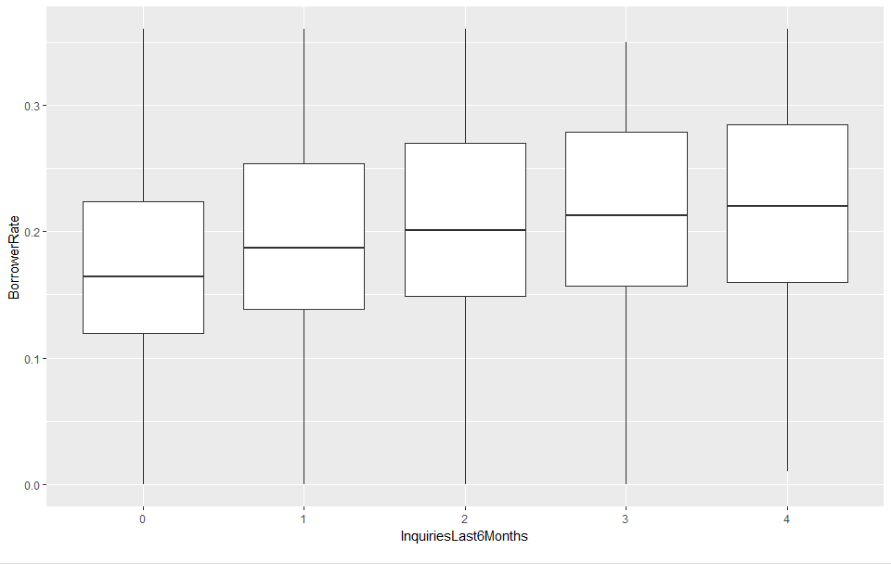结论:征信查询的次数越多,贷款的利率越高

4.2.7贷款利率和负债收益比的关系

``` ggplot(aes(x = DebtToIncomeRatio, y = BorrowerRate),
data = subset(loandata, loandata\$DebtToIncomeRatio <
quantile(loandata\$DebtToIncomeRatio,
probs = 0.95, "na.rm" = TRUE))) +
geom_point(alpha = 0.05, position = "jitter") +
stat_smooth(color = "red")```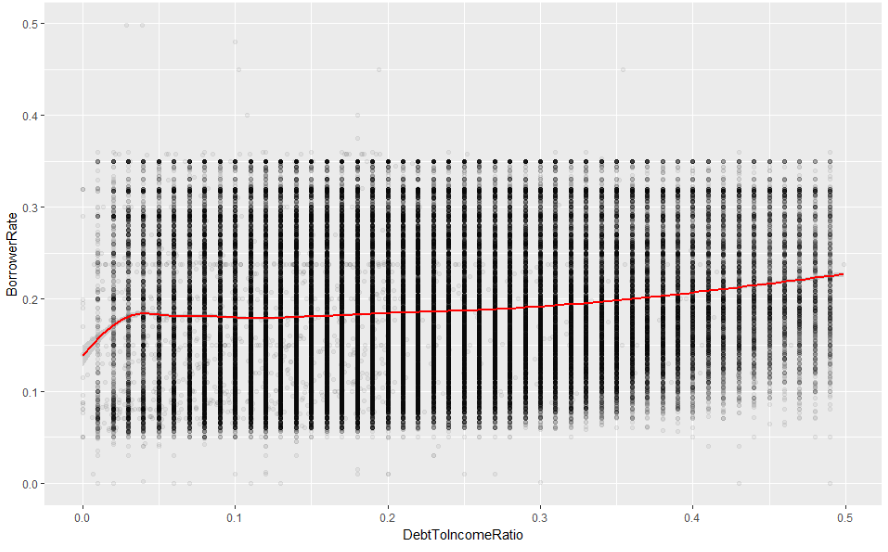结论:借款人的收入负债比越高，贷款利率也越高

4.2.8贷款利率和信用评分的关系

``` ggplot(aes(x = CreditScore, y = BorrowerRate), data = loandata) +
geom_point(alpha = 0.05) +
facet_wrap(~Phase, ncol = 1) +
coord_cartesian(xlim = c(400, 900), ylim = c(0, 0.4)) +
geom_line(stat = "summary", fun.y = mean) +
geom_line(stat = "summary", fun.y = median, linetype = 2, color = "red") +
geom_vline(xintercept = 600, color = "red")```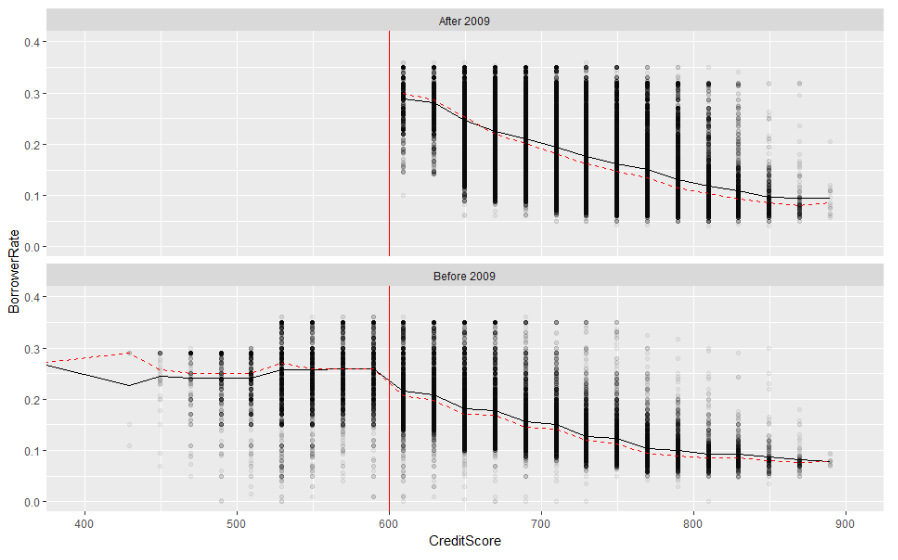结论:在2009年之前,Prosper还会为消费信用评级低于600分的借款人提供服务,在2009年重新上线之后,不再为评分低于600分的客户服务

4.2.9贷款利率和信用等级的关系

```# 2009年7月1日以前
ggplot(aes(x = CreditGrade, y = BorrowerRate),
data = subset(loandata, LoanOriginationDate < "2009-07-01")) +
scale_x_discrete(limits = c("HR", "E", "D", "C", "B", "A", "AA")) +
geom_boxplot() +
stat_summary(fun.y = mean, geom = "point", shape = 4) +
stat_summary(fun.y = mean, geom = "smooth", aes(group = 1))

# 2009年7月1日以后
ggplot(aes(x = ProsperRating..Alpha., y = BorrowerRate),
data = subset(loandata, LoanOriginationDate > "2009-07-01")) +
geom_boxplot() +
scale_x_discrete(limits = c("HR", "E", "D", "C", "B", "A", "AA")) +
stat_summary(fun.y = mean, geom = "point", shape = 4) +
stat_summary(fun.y = mean, geom = "smooth", aes(group = 1))```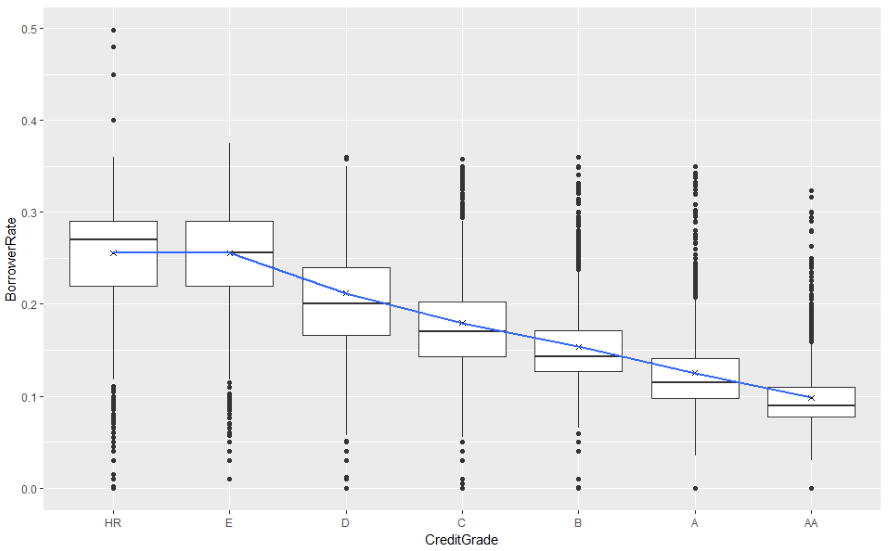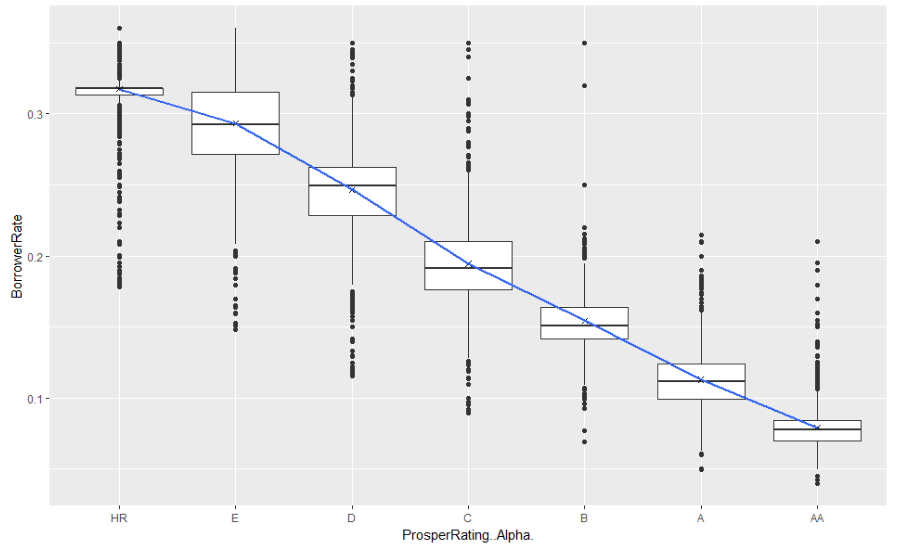结论:通过比较2009年7月1日前后贷款利率与借款人信用评级之间的关系发现，2009年7月1日后图形的均值连线明显斜率更大，说明Prosper在涉诉恢复运营后更为重视借款人的信用评级，评级对贷款　　　　　　　　　　  利率的影响权重更大了。

总结论:

```通过探索贷款利率与其他变量之间的关系，大致得出以下结论：

1. 贷款利率与过去7年的违约次数、过去6个月征信查询次数及负债收入比呈现出正相关关系。

2. 贷款利率似乎与借款人的月收入水平关系不明确。

3. 拥有房屋的借款人相比未拥有房屋的借款人更能够以较低利率筹得资金。

4. Prosper平台在2009年7月1日后似乎增加了信用评级对贷款利率的影响权重，并且不再对消费信用评分低于600分的借款人提供服务。```

4.3多变量分析

4.3.1贷款日期和贷款利率和贷款状态之间的关系

``` ggplot(aes(x = ListingCreationDate, y = BorrowerRate, color = LoanStatus), data = loandata) +
geom_point(alpha = 0.05) +
guides(colour = guide_legend(override.aes = list(alpha = 1, size = 5)))```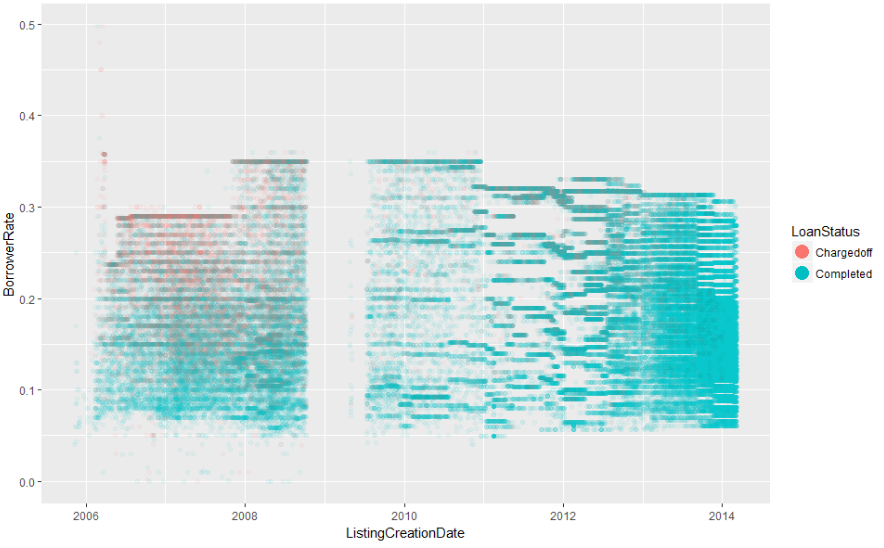结论:2009年之前的违约数远大于2009年之后,且利息越高违约率就越大

4.3.2贷款利率与消费信用评分及贷款状态的关系

``` ggplot(aes(x = CreditScore, y = BorrowerRate, color = LoanStatus),
data = loandata) +
geom_point(alpha = 0.05, position = "jitter") +
coord_cartesian(xlim = c(400, 900), ylim = c(0, 0.4)) +
facet_wrap(~Phase, ncol = 1) +
guides(color = guide_legend(override.aes = list(alpha = 1, size = 5)))```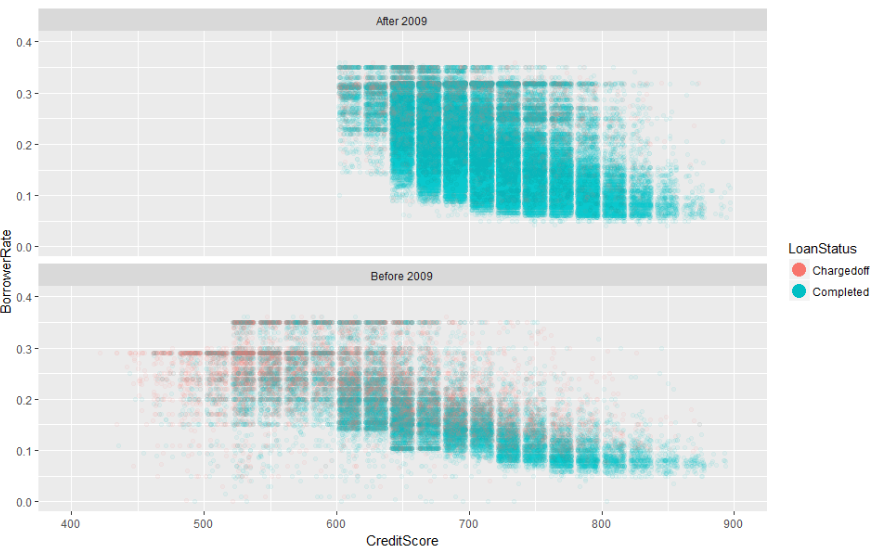结论:在2009年之前的债务违约明显很多,并且是以信用评分较低的且贷款利率较高客户为主,2009年之后平台不再为信用评分低于600的客户提供贷款,贷款的质量有了明显提升

也可以看出信用评分越高的客户,贷款的利率越低

4.3.3贷款利率与贷款总额及信用评级的关系

``` # 2009年7月1日之前
ggplot(aes(x = LoanOriginalAmount, y = BorrowerRate, color = CreditGrade),
data = subset(loandata, LoanOriginationDate < "2009-07-01" & CreditGrade != "NC")) +
geom_point(alpha = 0.5, position = "jitter") +
scale_color_brewer(type = "seq",
guide = guide_legend(title = "CreditGrade", reverse = F,
override.aes = list(alpha = 1, size = 5))) +
facet_wrap(~LoanStatus, ncol = 1)

# 2009年7月1日之后
ggplot(aes(x = LoanOriginalAmount, y = BorrowerRate, color = ProsperRating..Alpha.),
data = subset(loandata, LoanOriginationDate > "2009-07-01" &
!is.na(ProsperRating..Alpha.))) +
geom_point(alpha = 0.5) +
scale_color_brewer(type = "seq",
guide = guide_legend(title = "ProsPerRating", reverse = F,
override.aes = list(alpha = 1, size = 5))) +
facet_wrap(~LoanStatus, ncol = 1)```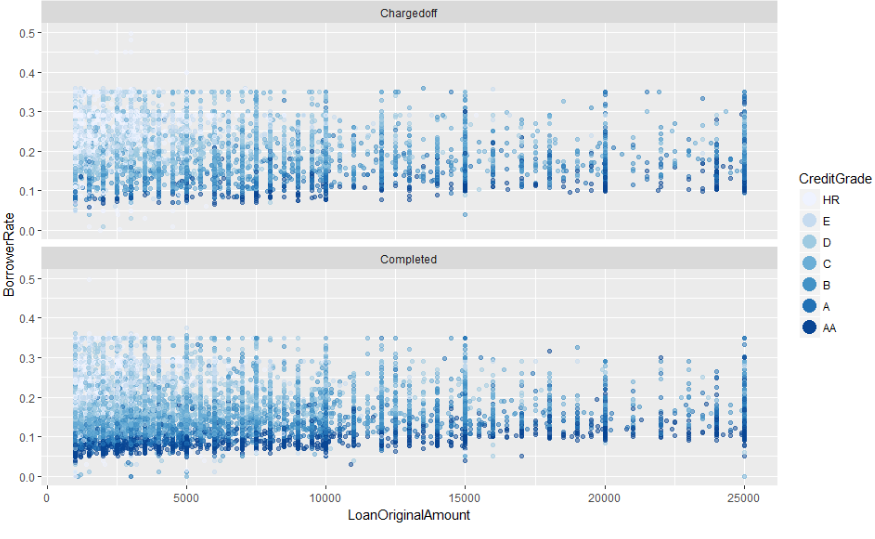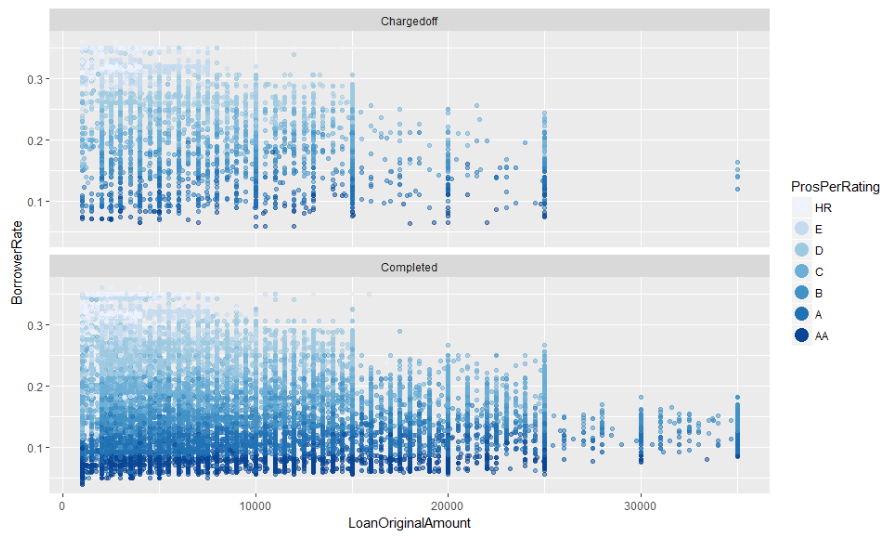结论:在2009年7月1日之后,Prosper将最大的贷款金额有25000提升到了35000,并且只针对信用评级为B以上的客户,随着贷款的金额越高利率在逐渐降低,此外平台的违约贷款都是由HR和E等级的客户导　　　　　　　　　　 致的

4.3.4贷款利率与贷款总额与信用卡使用频率的关系

``` # 2009年7月1日之前
ggplot(aes(x = LoanOriginalAmount, y = BorrowerRate, color = BankCardUse),
data = subset(loandata, LoanOriginationDate < "2009-07-01" &
!is.na(BankCardUse))) +
geom_point(alpha = 0.5) +
scale_color_brewer(type = "qual",
guide = guide_legend(title = "BankCardUse", reverse = T,
override.aes = list(alpha = 1, size = 5))) +
facet_wrap(~LoanStatus, ncol = 1)

# 2009年7月1日之后
ggplot(aes(x = LoanOriginalAmount, y = BorrowerRate, color = BankCardUse),
data = subset(loandata, LoanOriginationDate > "2009-07-01" &
!is.na(BankCardUse))) +
geom_point(alpha = 0.5) +
scale_color_brewer(type = "div",
guide = guide_legend(title = "BankCardUse", reverse = T,
override.aes = list(alpha = 1, size = 5))) +
facet_wrap(~LoanStatus, ncol = 1)```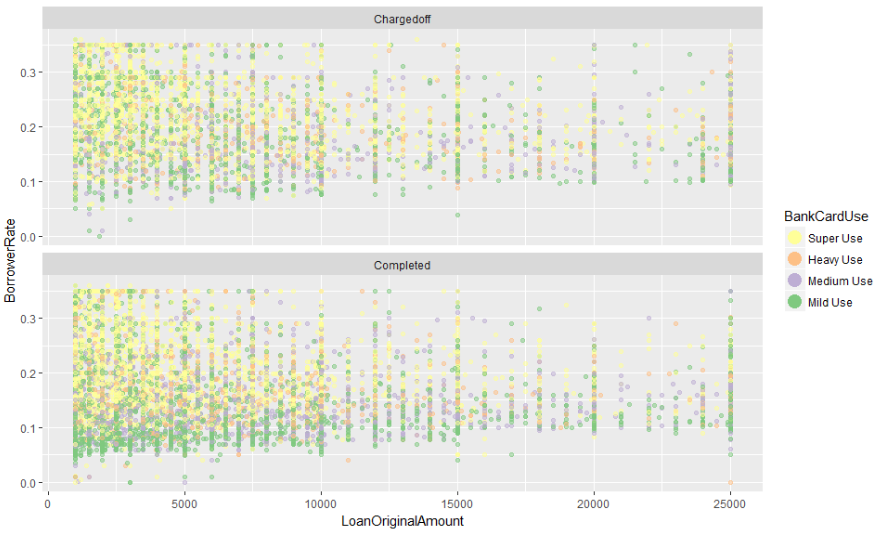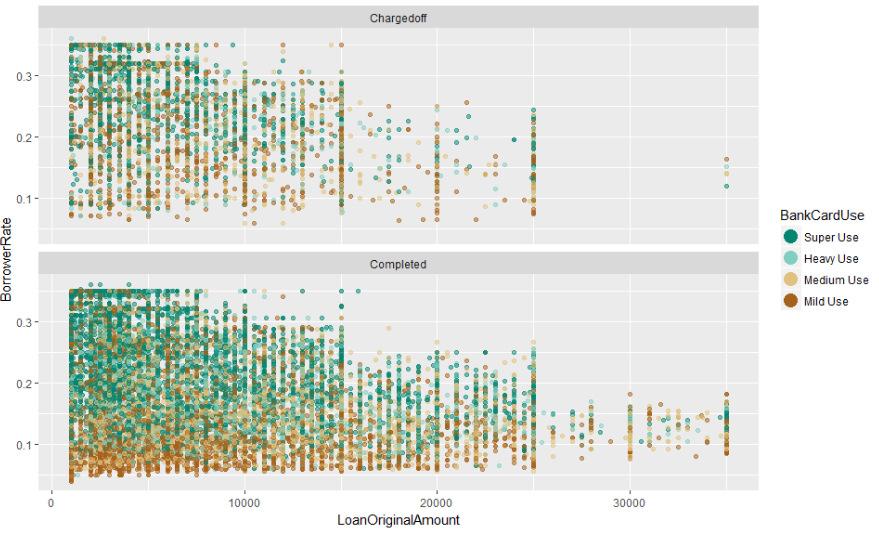结论:从信用卡的使用情况来看，时间节点前后并无明显变化。但是，违约贷款中，信用卡使用情况为Mild Use的借款人占比极低；相对的，信用卡使用情况为Super Use的客户占比却极高，这也印证了　　　　　　　　　　 前文的判断，信用卡的超额透支会对借款人的还款能力产生较大的负面影响。

总结论:

```本部分通过分时间节点、分贷款状态更为深入的探索了多重变量之间的关系，发现如下特点：

1. 2009年以前贷款违约数量明显高于2009年以后的数量，且利率越高，违约率越高！

2. 消费信用评分越高的借款人，其贷款利率也相对越低。

3. 2009年以前，Prosper限制了最大借款金额为25000美元，而在2009年以后放宽限制至35000美元，且似乎只针对信用评级B级以上的客户开放申请。并且随着贷款金额的增加，贷款利率在逐渐降低。

4. 信用评级为“HR”和“E”的借款人在违约组中的比例明显较高。

5. 信用卡使用情况为Super Use的借款人在违约组中的比例明显较高，使用情况为Mild Use的借款人在违约组中的占比极低。```

5.拟合因子,验证相关性

```# 2009年7月1日之前
m1 <- lm(I(BorrowerRate)~I(CreditScore),
data=subset(loan_data,
loan_data\$LoanOriginationDate <= '2009-07-01'
& !is.na(CreditScore)
& !is.na(LoanOriginalAmount)
& !is.na(BankCardUse)))
m2 <- update(m1,~ . + CreditGrade)
m3 <- update(m2,~ . + LoanOriginalAmount)
m4 <- update(m3,~ . + BankCardUse)
mtable(m1,m2,m3,m4)
# 2009年7月1日之后
m5 <- lm(I(BorrowerRate)~I(CreditScore),
data=subset(loan_data,
loan_data\$LoanOriginationDate > '2009-07-01'
& !is.na(CreditScore)
& !is.na(ProsperRating..Alpha.)
& !is.na(LoanOriginalAmount)
& !is.na(BankCardUse)))
m6 <- update(m5,~ . + ProsperRating..Alpha.)
m7 <- update(m6,~ . + LoanOriginalAmount)
m8 <- update(m7,~ . + BankCardUse)
mtable(m5,m6,m7,m8)```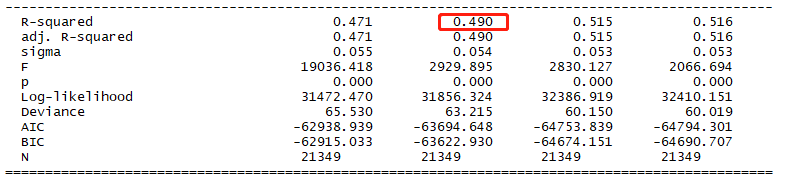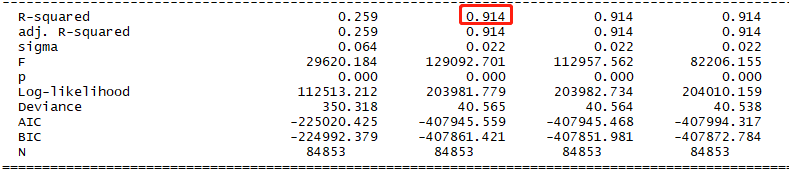结论:从表上看出2者的P值都是0说明拟合的较好,在2009年7月1日之前的R²是0.5左右,2009年7月1日之后的R²是0.9左右,说明平台降低了对信用评分的权重,加强信用等级的权重

## R语言-来自Prosper的贷款数据探索的更多相关文章

1. R语言-来自拍拍贷的数据探索

案例分析:拍拍贷是中国的一家在线借贷平台,网站撮合了一些有闲钱的人和一些急用钱的人.用户若有贷款需求,可在网站上选择借款金额. 本项目拟通过该数据集的探索,结合自己的理解进行分析,最终目的的是初步预测 ...

2. 【R笔记】R语言进阶之4：数据整形（reshape）

R语言进阶之4:数据整形(reshape) 2013-05-31 10:15 xxx 网易博客 字号:T | T 从不同途径得到的数据的组织方式是多种多样的,很多数据都要经过整理才能进行有效的分析,数 ...

3. 写论文，没数据？R语言抓取网页大数据

写论文,没数据?R语言抓取网页大数据 纵观国内外,大数据的市场发展迅猛,政府的扶持也达到了空前的力度,甚至将大数据纳入发展战略.如此形势为社会各界提供了很多机遇和挑战,而我们作为卫生(医学)统计领域的 ...

4. R语言进阶之4：数据整形（reshape）

一.通过重新构建数据进行整形 数据整形最直接的思路就把数据全部向量化,然后按要求用向量构建其他类型的数据.这样是不是会产生大量的中间变量.占用大量内存?没错.R语言的任何函数(包括赋值)操作都会有同样 ...

5. 大数据基础--R语言（刘鹏《大数据》课后习题答案）

1.R语言是解释性语言还是编译性语言?   解释性语言 2.简述R语言的基本功能.   R语言是一套完整的数据处理.计算和制图软件系统,主要包括以下功能: (1)数据存储和处理功能,丰富的数据读取与存 ...

6. R语言操作mysql上亿数据量(ff包ffbase包和ETLUtils包)

平时都是几百万的数据量,这段时间公司中了个大标,有上亿的数据量. 现在情况是数据已经在数据库里面了,需要用R分析,但是完全加载不进来内存. 面对现在这种情况,R提供了ff, ffbase , ETLU ...

7. 吴裕雄--天生自然 R语言开发学习：导入数据

2.3.6 导入 SPSS 数据 IBM SPSS数据集可以通过foreign包中的函数read.spss()导入到R中,也可以使用Hmisc 包中的spss.get()函数.函数spss.get() ...

8. 如何使用R语言解决可恶的脏数据

转自:http://shujuren.org/article/45.html 在数据分析过程中最头疼的应该是如何应付脏数据,脏数据的存在将会对后期的建模.挖掘等工作造成严重的错误,所以必须谨慎的处理那 ...

9. R语言学习笔记：取数据子集

上文介绍了,如何生成序列,本文介绍一下如何取出其数据子集 取出元素的逻辑值 > x<-c(0,-3,4,-1,45,90,5) > x>0  FALSE FALSE  T ...

## 随机推荐

1. Python 爬取美女图片，分目录多级存储

最近有个需求:下载https://mm.meiji2.com/网站的图片. 所以简单研究了一下爬虫. 在此整理一下结果,一为自己记录,二给后人一些方向. 爬取结果如图:   整体研究周期 2-3 天, ...

2. vue学习笔记（五）——指令

13条指令 1. v-text (数据绑定语法-插值) <span v-text="msg"></span> <!-- 和下面的一样 --> & ...

3. TCP socket 多线程 并发服务器（发送）与客户端（接收）

实现功能:Ubuntu上通过多线程实现服务器并发给客户端发送文件,携带包头,根据包头信息命名新文件.适用于短连接. 问题小结: 01. 调用嵌套在结构体中的结构体char 数组成员时,需要动态分配内存 ...

4. vue初学者

先分享一波福利 vue学习视频    链接:https://pan.baidu.com/s/1ggSfE75 密码:7h2a        1.先安装 webpack vue-cli          ...

5. sass和compass实战 读书笔记（一）

sass优势: 不做重复的工作 一  消除样式表冗余(通过变量赋值的方式) 1. 通过变量来复用属性值 2. 使用嵌套来快速写出多层级的选择器 3. 通过混合器来复用一段样式 4. 使用选择器继承来避 ...

6. 基于 HTML5 的 3D 工控隧道案例

隧道的项目我目前是第一次接触,感觉做起来的效果还蛮赞的,所以给大家分享一下.这个隧道项目的主要内容包括:照明.风机.车道指示灯.交通信号灯.情报板.消防.火灾报警.车行横洞.风向仪.COVI.微波车检 ...

7. JavaScript 教程：对象

JavaScript 对象是拥有属性和方法的数据.学过编程语言的都知道,此处不再详述! 1.对象的定义: <script> </script> 对象也可以先创建,再添加属性和属 ...

8. docker搭建私服

拉registry镜像 假设在192.168.100.17服务器上搭建私服 docker pull registry docker run -d -v /data/docker/registry:/v ...

9. 计算机改名引发的ORA

近期上班时,由于开机时老是提示" 局域网出现计算机重名冲突",于是把计算机名字给改了,从PC2010081312zeo改为了CXBIKKKKKKK,结果第二天来的时候,用PL/SQ ...

10. MySQL相关命令与备份

不加任何参数直接备份 mysqldump -uroot zabbix >/opt/zabbix.bak.sql 恢复,这样恢复时需要自已创建表 mysql -uroot < zabbix. ...